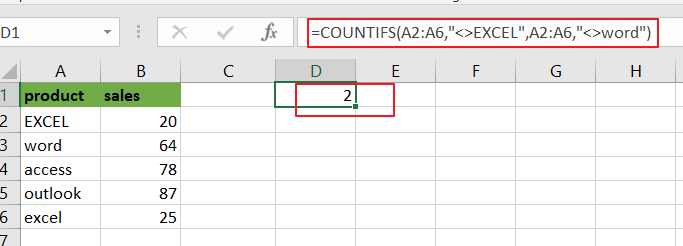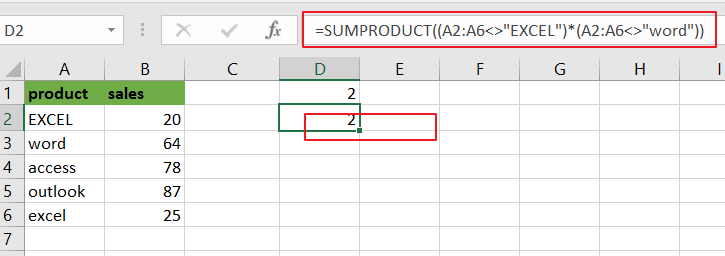# How to Count Cells Not equal to X or Y in Excel

This post will guide you how to count the number of cells not equal to criteria X or Y in a given range cells using a formula in Excel 2013/2016.You can easily to count cells equal to or not equal to a specific value through COUNTIF function. But if there is an easy way to count cells not equal to either one value or another value in a selected range of cells in Excel.

## Count Cells Not equals to Criteria X or Y

Assuming that you want to count cells that do not contain value “EXCEL”or “word” defined in a selected range(A1:B6).In this case, you can use the COUNTIFS function with multiple criteria to count cells not equal to “EXCEL” or “word”.

Enter the following formula in a blank cell, and press Enter key:

=COUNTIFS(A2:A6,”<>EXCEL”,A2:A6,”<>word”)Note: A2:A6 is the data range that you want to use. And both“EXCEL” and “word” are the text values that you want to count in range A2:A6. You need to change them as you need.

Now Let’s see how this formula works:

The COUNTIFS function will count the number of cells in a given range that match supplied criteria. All criteria must match while counting. In the above formula, there are two criteria. One criteria is “<>EXCEL”, it means if cell is not equal to “, another is “<>word”, it means that cells should not equal to “word”.

You can also use another array formula to achieve the same result of counting cells not equal to either “EXCEL” or “word” in A2:A6. Just using the following formula based on the SUMPRODUC function:

=SUMPRODUCT((A2:A6<>”EXCEL”)*(A2:A6<>”word”))### Related Functions

• Excel SUMPRODUCT function
The Excel SUMPRODUCT function multiplies corresponding components in the given one or more arrays or ranges, and returns the sum of those products. The syntax of the SUMPRODUCT function is as below:= SUMPRODUCT (array1,[array2],…)…
• Excel COUNTIFS function
The Excel COUNTIFS function returns the count of cells in a range that meet one or more criteria. The syntax of the COUNTIFS function is as below:= COUNTIFS(criteria_range1, criteria1, [criteria_range2, criteria2]…)…